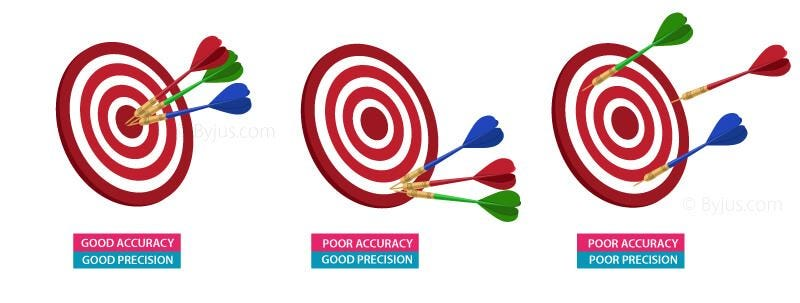# If not accuracy then

Original article was published on Deep Learning on MediumIn machine learning, the performance of a model is one of important factor for accurate prediction. For example if a models accuracy is 95% then the model’s predict would be accurate no?. Ahhh… that not the actual case no. If you consider a problem of classifying the images of cats and dogs, where there are 4,000 cat images and 3,000 dog images and if a model classifies them with an accuracy of 95%, then the model performs good. Consider a situation where you have to identify fraud bank transactions, out of 100,000 transactions only 2 or 3 will be fraudulent. If our model says all of the transactions are legit then the accuracy of the model will be above 99%. Will it be a good model? No…

Are you trying to say that accuracy cannot be used to measure the performance of the model? The fact is, for scenarios where the classes are nearly evenly distributed, like the cat and dog classification where both classes have nearly same number of data, accuracy will be a good measure for the performance of the model, in scenarios where the data for different class are greatly different, accuracy will not be a good measure for model’s performance. These type of scenarios are known as class imbalance.

If not accuracy then what? There are some other measures, recall, precision, f1 score which can be used along with accuracy to measure the performance of a model. These measure can also be used with scenarios where the classes are balanced. Before looking into these measures we have to get familiar with some terminologies.

In the figure above the prediction stands for whether the output of the model is yes or no and actual stands for real scenario whether yes or no. There are can be four different type of situations based on models output. If we consider the fraudulent transaction classifier, input being non fraudulent and the model predicting it as non fraudulent, input being non fraudulent and the model predicting it as fraudulent, input being fraudulent and the model predicting it as non fraudulent and input being fraudulent and the model predicting it as fraudulent. The first case is known as TrueNegative. In order to easily remember this you can think it as the the prediction made as Negative is true. Similarly the second case will be FalsePostive where the predicted positive is false, the third case FalseNegative where predicted as negative is false and the last one TruePostive where predicted as positive is true. The structure shown in the above figure is known as confusion matrix. Confusion matrix gives us the number of records for all the four cases.

How this confusion matrix will help us to get an accurate measure of model performance? Consider the bank transactions again, if the model works well, it should be able to identify the fraudulent transactions from legit transactions. i.e it should be able to identify most of the fraudulent transaction. That means \frac{correctly identified fraudulent transaction}{fraudulent transactions} should be high. If we translate the above expression in terms of the newly learnt technology. \frac{TruePostive}{(TruePostive+FalseNegative)}. This is known as recall. Recall stands for ratio between the correctly identified positives and total positives. In the bank transaction scenario it stand for the ratio between number of correctly classified fraudulent records and number of fraudulent records. That means if the recall is high then the model will ability of the model to correctly identify fraudulent transaction is also high.

Does having higher recall means the model’s performance is good? Considering the scenario of fraudulent transaction classification having a higher recall is good. But there can be another catch, consider the situation where all of the bank transaction are being classified as fraudulent. Then as per the recall equation the recall will be high. Does classifying all the transaction as fraudulent helps? No..

What can we do then? We have to pay attention towards the percentage of records being wrongly classified. i.e the model will falsely classify every record as Positive to increase the recall. So attention should be paid for the records which are wrongly classified as positive in other words FalsePositive. \frac{number of correctly classified fraudulent records}{number of records classified as fraudulent}. This measure will decrease if the model wrongly classifies legit transaction as fraudulent. The measure can be defined in other words as \frac{TruePostive}{(TruePositive + FalsePositive)}, which is called as precision. Precision measures the percentage of correctly labeled positives out of the total positives. As in the above mentioned scenario if the model tries to classify all the transactions as fraudulent to keep the recall high, then the precision will go down.

The relationships between precision, recall and accuracy and other measures like f1 score and others will be covered in the next article.